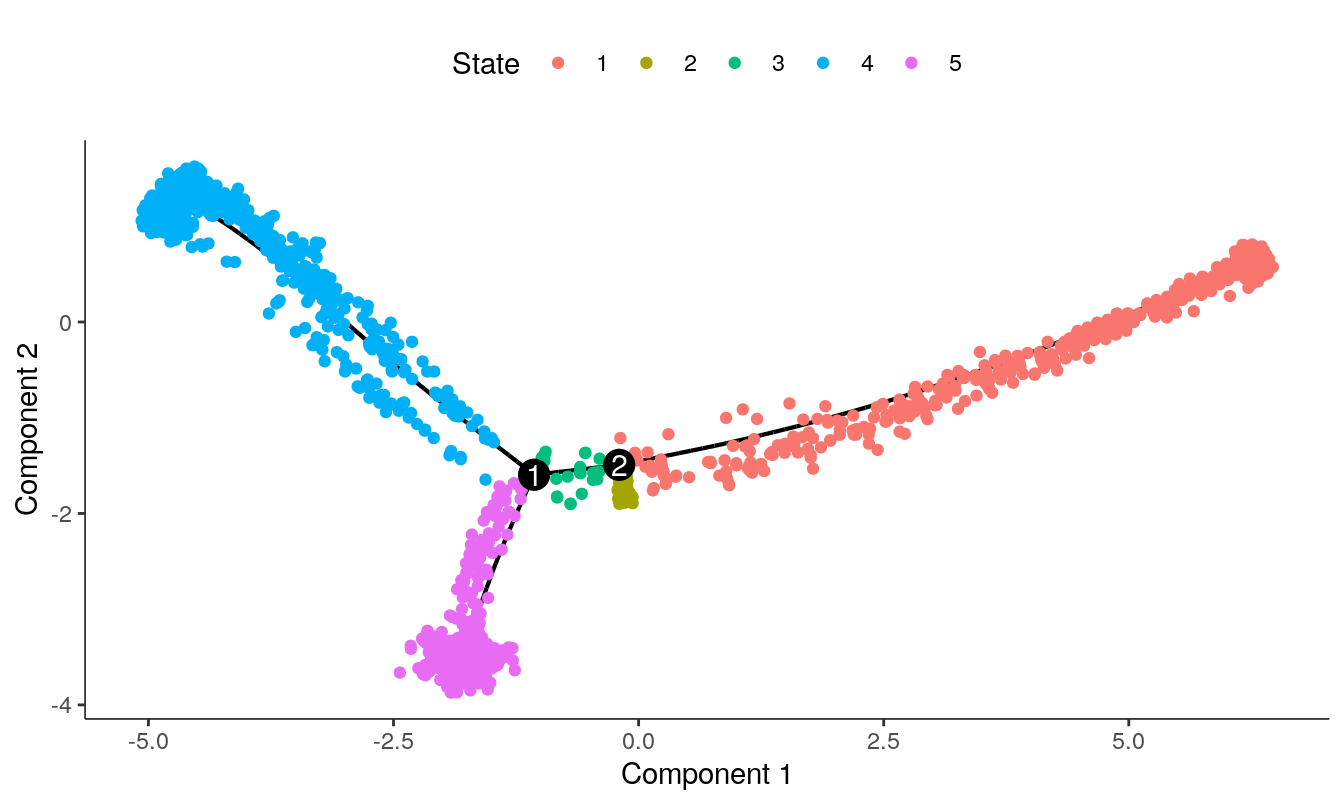Introduction

tradeSeq is an R package that allows analysis of gene expression along trajectories. While it has been developed and applied to single-cell RNA-sequencing (scRNA-seq) data, its applicability extends beyond that, and also allows the analysis of, e.g., single-cell ATAC-seq data along trajectories or bulk RNA-seq time series datasets. For every gene in the dataset, tradeSeq fits a generalized additive model (GAM) by building on the mgcv R package. It then allows statistical inference on the GAM by assessing contrasts of the parameters of the fitted GAM model, aiding in interpreting complex datasets. All details about the tradeSeq model and statistical tests are described in our preprint (Van den Berge et al. 2019).

In this vignette, we analyze a subset of the data from (Paul et al. 2015). A SingleCellExperiment object of the data has been provided with the tradeSeq package and can be retrieved as shown below. The data and UMAP reduced dimensions were derived from following the Monocle 3 vignette.

The main vignette focuses on using tradeSeq downsteam of slingshot. Here, we present how to use tradeSeq downstream of monocle(Qiu et al. 2017).

library(RColorBrewer)
library(SingleCellExperiment)
library(monocle)

# For reproducibility
palette(brewer.pal(8, "Dark2"))
counts <- as.matrix(countMatrix)
rm(countMatrix)

Fit trajectories using Monocle

We will fit developmental trajectories using the monocle package.

set.seed(200)
pd <- data.frame(cells = colnames(counts), cellType = celltype)
rownames(pd) <- pd$cells fd <- data.frame(gene_short_name = rownames(counts)) rownames(fd) <- fd$gene_short_name
cds <- newCellDataSet(counts, phenoData = new("AnnotatedDataFrame", data = pd),
featureData = new("AnnotatedDataFrame", data = fd))
cds <- estimateSizeFactors(cds)
cds <- reduceDimension(cds, max_components = 2)
cds <- orderCells(cds)
cds <- orderCells(cds, root_state = 5)
plot_cell_trajectory(cds)Fit Smoothers

Only possible for DDRTree. There are two options to do this. Either use the extract_monocle_info function and fit using the default fitGAM mode.

info <- extract_monocle_info(cds)
sce <- fitGAM(counts = Biobase::exprs(cds),
cellWeights = info$cellWeights, pseudotime = info$pseudotime)

or use the specific CellDataSet method.

sce <- fitGAM(cds, verbose = TRUE)

You can then analyse the sce object following the main vignette.

Monocle3

As of now (06/2020), monocle3(Cao et al. 2019), is still in its beta version. Therefore, we have no plan yet to include a S4 method for monocle3 while it is not on CRAN or Bioconductor and the format is still moving. However, we present below a way to use tradeSeq downstream of monocle3 as of version ‘0.2’, for a fully connected graph. We follow the tutorial from the monocle3 website.

Constructing the trajectory

You will need to install monocle3 from here before running the code below.

set.seed(22)
library(monocle3)
# Create a cell_data_set object
cds <- new_cell_data_set(counts, cell_metadata = pd,
row.names = rownames(counts)))
# Run PCA then UMAP on the data
cds <- preprocess_cds(cds, method = "PCA")
cds <- reduce_dimension(cds, preprocess_method = "PCA",
reduction_method = "UMAP")

# First display, coloring by the cell types from Paul et al
plot_cells(cds, label_groups_by_cluster = FALSE, cell_size = 1,
color_cells_by = "cellType")

# Running the clustering method. This is necessary to the construct the graph
cds <- cluster_cells(cds, reduction_method = "UMAP")
# Visualize the clusters
plot_cells(cds, color_cells_by = "cluster", cell_size = 1)

# Construct the graph
# Note that, for the rest of the code to run, the graph should be fully connected
cds <- learn_graph(cds, use_partition = FALSE)

# We find all the cells that are close to the starting point
cell_ids <- colnames(cds)[pd$cellType == "Multipotent progenitors"] closest_vertex <- cds@principal_graph_aux[["UMAP"]]$pr_graph_cell_proj_closest_vertex
closest_vertex <- as.matrix(closest_vertex[colnames(cds), ])
closest_vertex <- closest_vertex[cell_ids, ]
closest_vertex <- as.numeric(names(which.max(table(closest_vertex))))
mst <- principal_graph(cds)$UMAP root_pr_nodes <- igraph::V(mst)$name[closest_vertex]

# We compute the trajectory
cds <- order_cells(cds, root_pr_nodes = root_pr_nodes)
plot_cells(cds, color_cells_by = "pseudotime")

Extracting the pseudotimes and cell weights for tradeSeq

library(magrittr)
# Get the closest vertice for every cell
y_to_cells <-  principal_graph_aux(cds)$UMAP$pr_graph_cell_proj_closest_vertex %>%
as.data.frame()
y_to_cells$cells <- rownames(y_to_cells) y_to_cells$Y <- y_to_cells$V1 # Get the root vertices # It is the same node as above root <- cds@principal_graph_aux$UMAP$root_pr_nodes # Get the other endpoints endpoints <- names(which(igraph::degree(mst) == 1)) endpoints <- endpoints[!endpoints %in% root] # For each endpoint cellWeights <- lapply(endpoints, function(endpoint) { # We find the path between the endpoint and the root path <- igraph::shortest_paths(mst, root, endpoint)$vpath[]
path <- as.character(path)
# We find the cells that map along that path
df <- y_to_cells[y_to_cells$Y %in% path, ] df <- data.frame(weights = as.numeric(colnames(cds) %in% df$cells))
colnames(df) <- endpoint
return(df)
}) %>% do.call(what = 'cbind', args = .) %>%
as.matrix()
rownames(cellWeights) <- colnames(cds)
pseudotime <- matrix(pseudotime(cds), ncol = ncol(cellWeights),
nrow = ncol(cds), byrow = FALSE)
sce <- fitGAM(counts = counts,
pseudotime = pseudotime,
cellWeights = cellWeights)

Then, the sce object can be analyzed following the main vignette.

Session

sessionInfo()
## R version 4.0.3 (2020-10-10)
## Platform: x86_64-pc-linux-gnu (64-bit)
## Running under: Ubuntu 18.04.5 LTS
##
## Matrix products: default
## BLAS:   /home/biocbuild/bbs-3.12-bioc/R/lib/libRblas.so
## LAPACK: /home/biocbuild/bbs-3.12-bioc/R/lib/libRlapack.so
##
## locale:
##   LC_CTYPE=en_US.UTF-8       LC_NUMERIC=C
##   LC_TIME=en_US.UTF-8        LC_COLLATE=C
##   LC_MONETARY=en_US.UTF-8    LC_MESSAGES=en_US.UTF-8
##   LC_PAPER=en_US.UTF-8       LC_NAME=C
##  LC_MEASUREMENT=en_US.UTF-8 LC_IDENTIFICATION=C
##
## attached base packages:
##   splines   parallel  stats4    stats     graphics  grDevices utils
##   datasets  methods   base
##
## other attached packages:
##   monocle_2.18.0              DDRTree_0.1.5
##   irlba_2.3.3                 VGAM_1.1-4
##   ggplot2_3.3.2               Matrix_1.2-18
##   SingleCellExperiment_1.12.0 SummarizedExperiment_1.20.0
##   Biobase_2.50.0              GenomicRanges_1.42.0
##  GenomeInfoDb_1.26.0         IRanges_2.24.0
##  S4Vectors_0.28.0            BiocGenerics_0.36.0
##  MatrixGenerics_1.2.0        matrixStats_0.57.0
##  knitr_1.30
##
## loaded via a namespace (and not attached):
##   viridis_0.5.1          edgeR_3.32.0           viridisLite_0.3.0
##   GenomeInfoDbData_1.2.4 yaml_2.2.1             slam_0.1-47
##   ggrepel_0.8.2          pillar_1.4.6           lattice_0.20-41
##  glue_1.4.2             limma_3.46.0           densityClust_0.3
##  digest_0.6.27          XVector_0.30.0         colorspace_1.4-1
##  fastICA_1.2-2          htmltools_0.5.0        plyr_1.8.6
##  pkgconfig_2.0.3        pheatmap_1.0.12        HSMMSingleCell_1.9.0
##  qlcMatrix_0.9.7        zlibbioc_1.36.0        purrr_0.3.4
##  scales_1.1.1           RANN_2.6.1             Rtsne_0.15
##  BiocParallel_1.24.0    proxy_0.4-24           tibble_3.0.4
##  combinat_0.0-8         mgcv_1.8-33            farver_2.0.3
##  generics_0.0.2         docopt_0.7.1           ellipsis_0.3.1
##  withr_2.3.0            sparsesvd_0.2          pbapply_1.4-3
##  slingshot_1.8.0        magrittr_1.5           crayon_1.3.4
##  evaluate_0.14          nlme_3.1-150           FNN_1.1.3
##  tools_4.0.3            lifecycle_0.2.0        stringr_1.4.0
##  locfit_1.5-9.4         munsell_0.5.0          cluster_2.1.0
##  DelayedArray_0.16.0    compiler_4.0.3         rlang_0.4.8
##  grid_4.0.3             RCurl_1.98-1.2         igraph_1.2.6
##  labeling_0.4.2         bitops_1.0-6           rmarkdown_2.5
##  gtable_0.3.0           reshape2_1.4.4         R6_2.4.1
##  gridExtra_2.3          dplyr_1.0.2            ape_5.4-1
##  princurve_2.1.5        stringi_1.5.3          Rcpp_1.0.5
##  vctrs_0.3.4            tidyselect_1.1.0       xfun_0.18